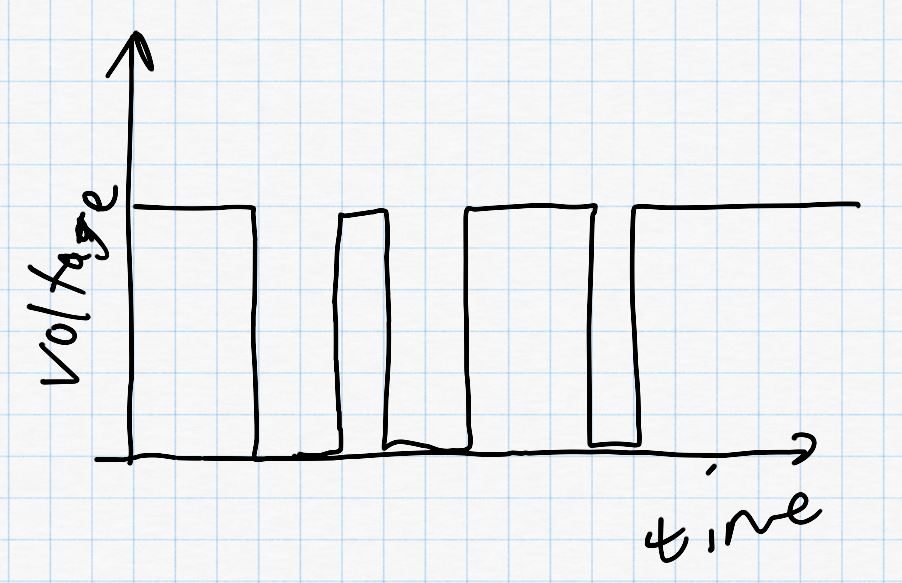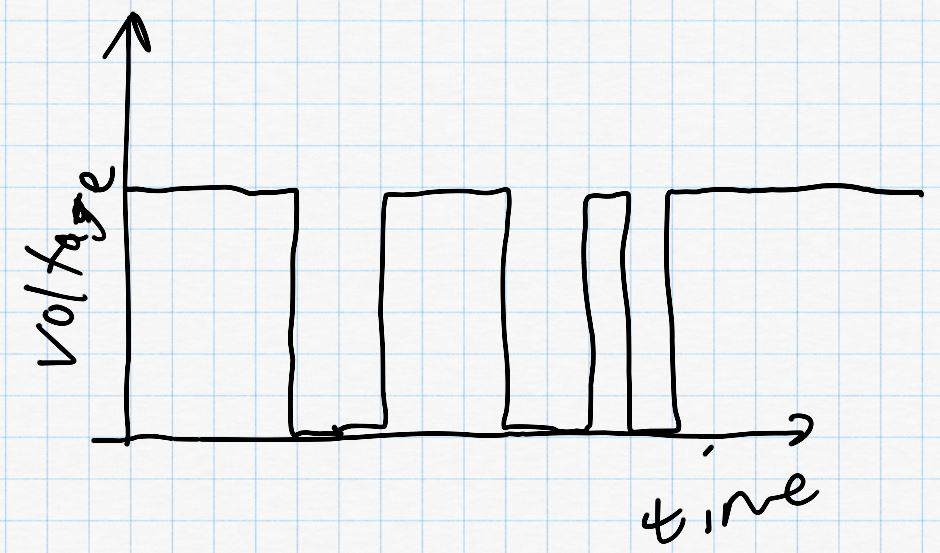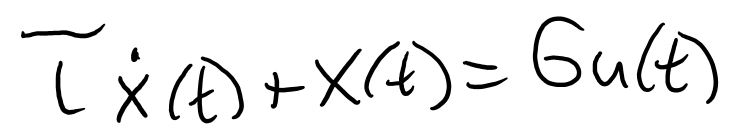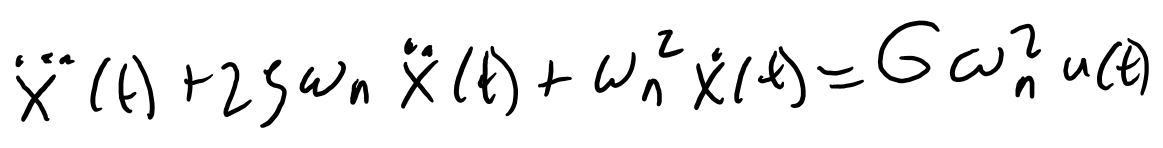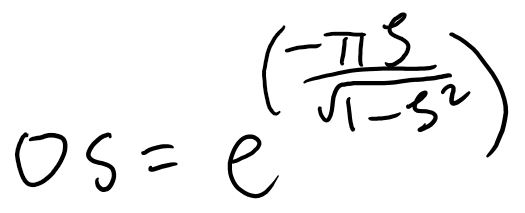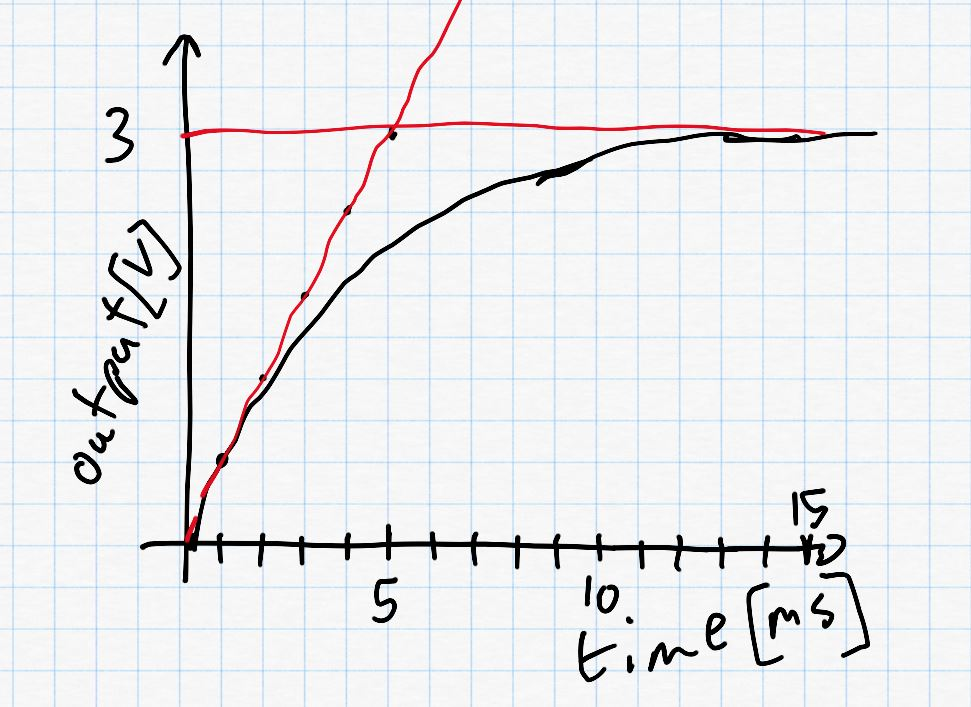Robotics 1
UART Communication
QuizQuestion 2:  What do we mean by referring to communication as 'serial' communication?
Question 3:  What are the units of 'baud rate'?
Question 4:  Suppose that I want to send the number 114 over a UART communication.  Which of these voltage vs. time plots is correct?
Question 5:  Suppose that you have set the baud rate to 57600.  How much time in milliseconds does it take to send one bit?  Round your answer to three places after the decimal, and don't enter units.
Question 6:  If you have set the baud rate to 57600, how much time (in milliseconds) will it take to send one 8-bit byte, given that there is one start bit and one stop bit?  Round your answer to three places after the decimal, and don't enter units.
Question 7:  Suppose that we have the voltage/time plot as shown here during our UART communication.  What is the number that is being sent?  Your answer should be an integer.
Question 8:  Which of these is a rule for how UART works?
Question 9:  In ASCII, what decimal number is associated with the question mark character '?'
Question 10:  What is the largest value you can send over UART in a single byte?
Question 11:  Which of these equations represents the relationship between the input signal and the output signal for a first-order system?
Question 12:  Which of these equations represents the relationship between the input signal and the output signal for a second-order system?
Question 13:  Suppose you have captured a step response that looks like this.  What is the value of the time constant, tau, in units of seconds?
Question 14:  Suppose you have captured a step response that looks like this.  What is the value of the damping ratio, zeta?
Question 15:  For the step response in Question 14, what is the value of the natural frequency, omega n, in units of radians per second?
Question 1: What does 'UART' stand for?
Universal Application for Receiving Transmissions
Understandable Applications for Robotics Topography
Underwater Anchors for Robotic Transmissions
'Serial' communication means that we are sending a series of bytes to make a larger number
'Serial' communication means that we send bits all at the same time, rather than one after the other
'Serial' communication means that we send bits one after the other, rather than all at the same time
'Serial' communication means that we are sending data about serial manipulators
Volts per bit
Bits per second
Bytes per second
The left-most bit is sent first
The right-most bit is sent first
The middle bit is sent first
The fourth bit is sent first
Volts per byte
255
-127
127
256